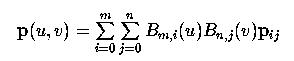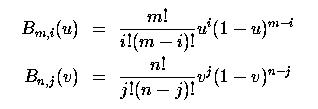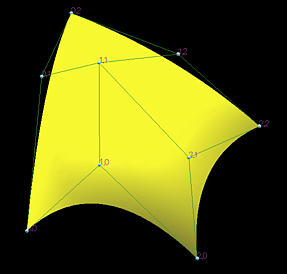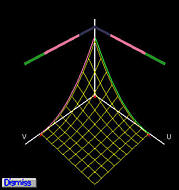# Bézier Surfaces: ConstructionA Bézier surface is defined by a two-dimensional set of control points pi,j, where i is in the range of 0 and m, and j is in the range of 0 and n. Thus, in this case, we have m+1 rows and n+1 columns of control points and the control point on the i-th row and j-th column is denoted by pi,j. Note that we have (m+1)(n+1) control points in total.

The following is the equation of a Bézier surface defined by m+1 rows and n+1 columns of control points:where Bm,i(u) and Bn,j(v) are the i-th and j-th Bézier basis functions in the u- and v- directions, respectively. Recall from the discussion of Bézier curves, these basis functions are defined as follows:Since Bm,i(u) and Bn,j(v) are degree m and degree n functions, we shall say this is a Bézier surface of degree (m,n). The set of control points is usually referred to as the Bézier net or control net. Note that parameters u and v are in the range of 0 and 1 and hence a Bézier surface maps the unit square to a rectangular surface patch.

The following figure shows a Bézier surface defined by 3 rows and 3 columns (i.e., 9) control points and hence is a Bézier surface of degree (2,2).### Basis Functions

The basis functions of a Bézier surface are the coefficients of control points. From the definition, it is clear that these two-dimensional basis functions are the product of two one-dimensional Bézier basis functions and consequently the basis functions for a Bézier surface are parametric surfaces of two variables u and v defined on the unit square. The following figures show the basis functions for control points p0,0 (left) and p1,1 (right), respectively. For control point p0,0, its basis function is the product of two one-dimensional Bézier basis functions B2,0(u) in the u direction and B2,0(v) in the v direction. In the left figure, both B2,0(u) and B2,0(v) are shown along with their product (shown in wireframe). The right figure shows the basis function for p1,1, which is the product of B2,1(u) in the u direction and B2,1(v) in the v direction.### Tensor Product Surfaces

The tensor product technique constructs surfaces by "multiplying" two curves. Given two Bézier, B-spline or NURBS curves, the tensor product method constructs a surface by multiplying the basis functions of the first curve with the basis functions of the second and use the results as the basis functions for a set of two-dimensional control points. Surfaces generated this way are called tensor product surfaces. Therefore, Bézier surfaces, B-spline surfaces and NURBS surfaces are all tensor product surfaces.